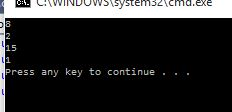# Arithmetic operator in C#

##### How to use arithmetic operator in c#?

Arithmetic operator's  are used calculate the operations like addition, substraction, multilication, division etc.

```  using System;
using System.Collections.Generic;
using System.Linq;
using System.Text;
class Program
{
static void Main()
{
int a = 5, b = 3;
Console.WriteLine(a + b);
Console.WriteLine(a - b);
Console.WriteLine(a * b);
Console.WriteLine(a / b);

}
}
```

In the above code snippet we have declared two variables a, b. In this arithmetic operator we are performing addition, substraction, multiplication and division operations. In the addition operator we are adding the two variables with the values a, b with the operator '+'. In the substraction operator we are substracting the two variables with '-' operator. In the multiplication operator we are adding two variables with the operator '*' operator. In the division operator we are dividing the two variables with the operator '/ '.

outputViews: 3100 | Post Order: 6